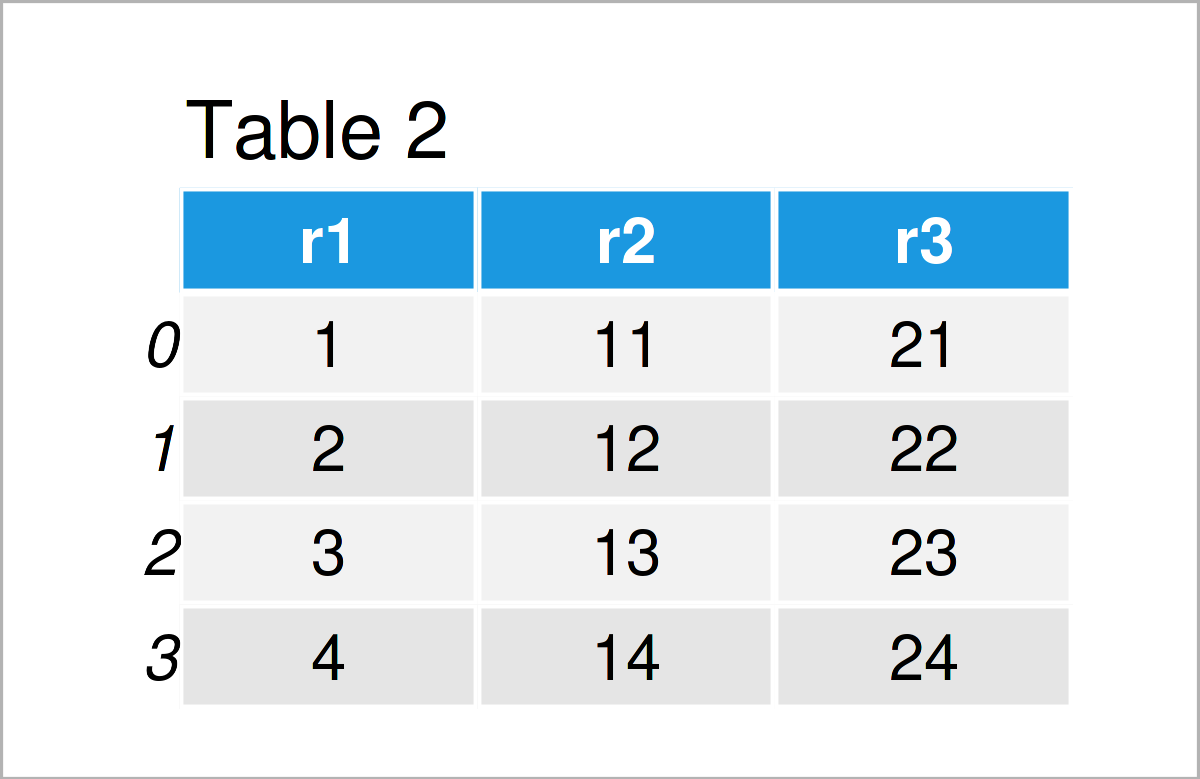# Convert NumPy Array to pandas DataFrame in Python (2 Examples)

This article demonstrates how to create a pandas DataFrame from a NumPy array in the Python programming language.

The table of content is structured as follows:

Let’s do this…

## Example Data & Add-On Libraries

To be able to use the functions of the NumPy library, we first need to import NumPy:

`import numpy as np                                # Load NumPy`

Furthermore, have a look at the following example array:

```my_array = np.array([[1, 2, 3, 4],                # Create NumPy array
[11, 12, 13, 14],
[21, 22, 23, 24]])
print(my_array)                                   # Print NumPy array
# [[ 1  2  3  4]
#  [11 12 13 14]
#  [21 22 23 24]]```

The previous output of the Python console shows that our NumPy array has three rows and four columns. We will use this array as a basis for the following examples.

## Example 1: Create pandas DataFrame from NumPy Array by Columns

Example 1 demonstrates how to convert a NumPy array to a pandas DataFrame by columns.

We first need to load the pandas library, if we want to use the corresponding functions:

`import pandas as pd                               # Import pandas library in Python`

In the next step, we can apply the DataFrame function of the pandas library to construct our pandas DataFrame.

Within the DataFrame function, we have to specify which values of our NumPy array should be stored in which column of the DataFrame:

```my_data1 = pd.DataFrame({'c1': my_array[:, 0],    # Create pandas DataFrame
'c2': my_array[:, 1],
'c3': my_array[:, 2],
'c4': my_array[:, 3]})
print(my_data1)                                   # Print pandas DataFrame```Have a look at the previous table. It shows that our NumPy array has been transformed to a pandas DataFrame that comprises three lines and four columns.

Each of the columns of the NumPy array has been converted to a column in the pandas DataFrame, i.e. the structure has been kept the same.

In the next example, I’ll show you a different way on how to convert a NumPy array to a pandas DataFrame. So keep on reading!

## Example 2: Create pandas DataFrame from NumPy Array by Rows

In Example 2, I’ll demonstrate how to convert each row of a NumPy array to a column of a new pandas DataFrame using the Python programming language.

To accomplish this, we can use the DataFrame function once again. However, this time, we have to specify different subsets of our NumPy array for each column of the new DataFrame:

```my_data2 = pd.DataFrame({'r1': my_array[0, :],    # Create pandas DataFrame
'r2': my_array[1, :],
'r3': my_array[2, :]})
print(my_data2)                                   # Print pandas DataFrame```As shown in Table 2, we have created another pandas DataFrame where each row of the NumPy array corresponds to one variable in the DataFrame.

## Video & Further Resources

Do you want to learn more about the creation of a pandas DataFrame from a NumPy array? Then you might have a look at the following video on my YouTube channel. I demonstrate the contents of this page in the video instruction.

In addition, you could have a look at the other articles on my website. A selection of articles can be found below:

In this Python programming tutorial you have learned how to construct a pandas DataFrame based on a NumPy array. If you have any additional questions, let me know in the comments section.

Subscribe to the Statistics Globe Newsletter